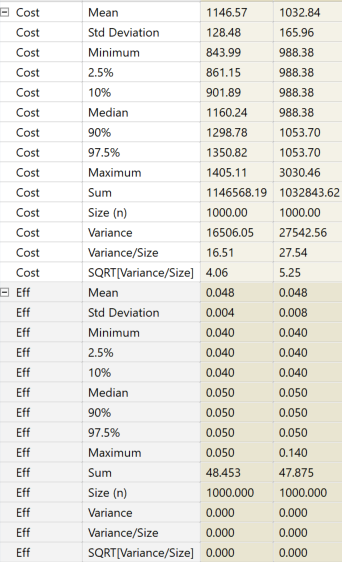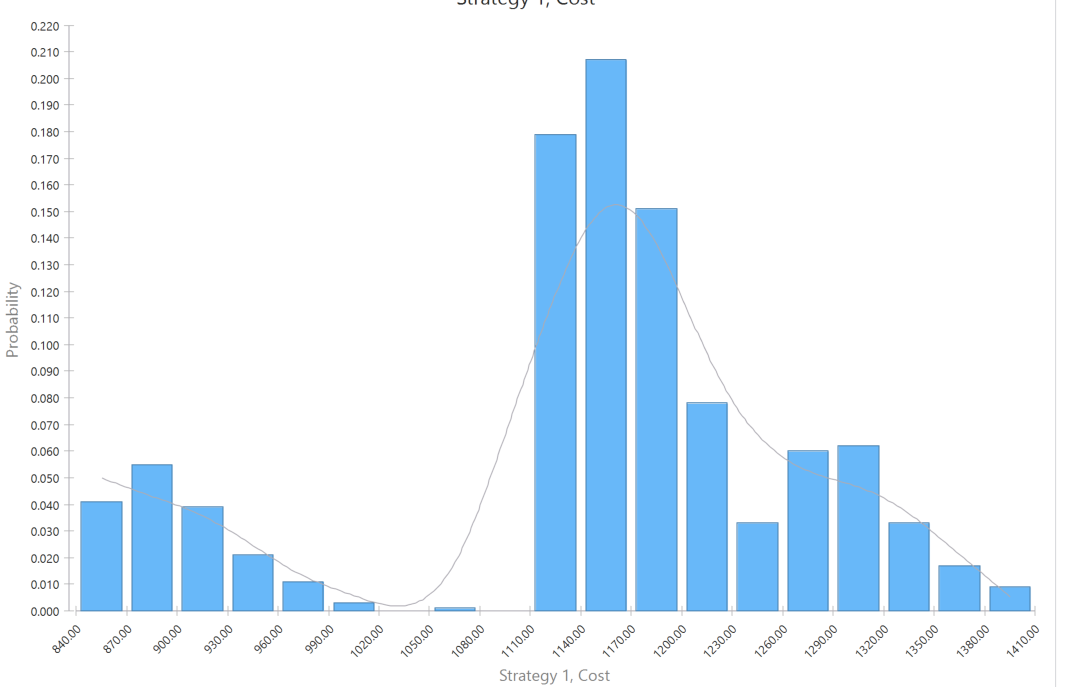# Converting results from a branch into a parameter

Hello everyone, .

In short, I have a branch of a microsim/parameter Markov model that I'd like to convert into a parameter so I can run sensitivity analyses and to increase its efficiency of the model. Is there an easy way to parameterize a Monte Carlo output or use TA2020 directly to convert the branch into a best-fit parameter?

Thank you!

Here's the Monte Carlo output I have for the branches:• Official comment

Thank you for an interesting question.  I have not thought of approaching reduction of a microsimulation process to a parametric function.  In general there are some risks involved in creating parameterizated representation that vary from overfitting to inappropriate extrapolation.

One question would be what would you consider to be tine input parameter(s) for the function to be.  For example is a single parameter alpha that varies from 0 to 1 and returns cost as function of alpha cost(alpha) in range 840 to 1410 and companion function ProbabilityOfCost(Cost(alpha)) which would have the shape of your empirical cost distribution.  If that is the case the problem is quite, using a simple linear equation for cost(alpha) and a table definition for ProbabilityOfCost( ).

However, you may have some other parameterization in mind, than what I have outlined above.  Could you elaborate on what functional relationships you are seeking and how would you want to use them in specific context of the target model.

• My costs aren't very easy to parameterize:• Thank you for your response! The branch utilizes a dirichlet distribution to determine which of 5 interventions (and their associated costs and QALY) that an individual will get. One problem I see with using a simple function, is that there is a chance of exiting the tree and going back into the tree (I guess you could model this with p*function+p^2*function+p^3*function...  (I could be confusing the formula here) but the function becomes very complex).

• Please be cautious about converting a Microsimulation model to a parametric function. Simulation models allow for individual patient history and characteristics to affect patients within a random walk through the model. I don't think there is any parametric distribution or formula that can replicate such a complex process.

In addition, interventions should be studied separately rather than sending patients randomly through each one. Running them separately is the only way to compare the results of all strategies for decision analysis.

• I agree with Andrew, that a simple Markov process can represent very complicated functional relationships.

Just as an analogy a simple iterative (or recursive) implementation of the factorial function n! can be approximated with different non-iterative functions but they are not simple.

https://en.wikipedia.org/wiki/Stirling%27s_approximation

You are likely to spend more time looking for the approximation, than running the model as is.  You would also have to run the model with and without the candidate approximations to convince yourself that you got it right.